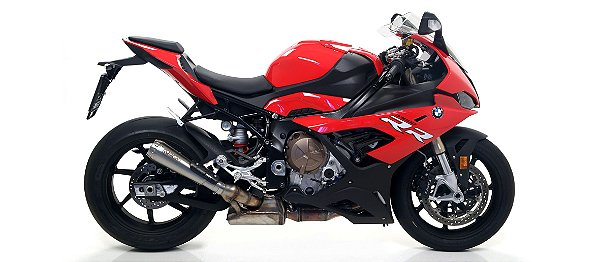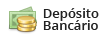# Ponteira Arrow Pro Race - BMW S 1000 RR 20'~

Código: 71903 Marca:
Selecione a opção de Cor:
Selecione a opção de Tipo de material:
Comprar Estoque: 60 dias úteis
6x de R\$ 721,83
ou R\$ 3.984,52 via Depósito
Comprar Estoque: 60 dias úteis
6x de R\$ 721,83
ou R\$ 3.984,52 via Depósito
Comprar Estoque: 60 dias úteis
6x de R\$ 721,83
A partir de R\$ 4.331,00
ou R\$ 3.984,52 via Depósito
• 1x de R\$ 4.977,00 sem juros
• 2x de R\$ 2.488,50 sem juros
• 3x de R\$ 1.659,00 sem juros
• 4x de R\$ 1.244,25 sem juros
• 5x de R\$ 995,40 sem juros
• 6x de R\$ 829,50 sem juros
• 1x de R\$ 4.977,00 sem juros
• 2x de R\$ 2.488,50 sem juros
• 3x de R\$ 1.659,00 sem juros
• 4x de R\$ 1.338,62
• 5x de R\$ 1.086,44
• 6x de R\$ 918,43
• 7x de R\$ 798,53
• 8x de R\$ 708,70
• 9x de R\$ 638,91
• 10x de R\$ 583,15
• 11x de R\$ 537,60
• 12x de R\$ 499,70
• R\$ 4.578,84• 1x de R\$ 4.331,00 sem juros
• 2x de R\$ 2.165,50 sem juros
• 3x de R\$ 1.443,66 sem juros
• 4x de R\$ 1.082,75 sem juros
• 5x de R\$ 866,20 sem juros
• 6x de R\$ 721,83 sem juros
• 1x de R\$ 4.331,00 sem juros
• 2x de R\$ 2.165,50 sem juros
• 3x de R\$ 1.443,66 sem juros
• 4x de R\$ 1.164,87
• 5x de R\$ 945,42
• 6x de R\$ 799,22
• 7x de R\$ 694,89
• 8x de R\$ 616,71
• 9x de R\$ 555,98
• 10x de R\$ 507,46
• 11x de R\$ 467,82
• 12x de R\$ 434,84
• R\$ 3.984,52• 1x de R\$ 4.331,00 sem juros
• 2x de R\$ 2.165,50 sem juros
• 3x de R\$ 1.443,66 sem juros
• 4x de R\$ 1.082,75 sem juros
• 5x de R\$ 866,20 sem juros
• 6x de R\$ 721,83 sem juros
• 1x de R\$ 4.331,00 sem juros
• 2x de R\$ 2.165,50 sem juros
• 3x de R\$ 1.443,66 sem juros
• 4x de R\$ 1.164,87
• 5x de R\$ 945,42
• 6x de R\$ 799,22
• 7x de R\$ 694,89
• 8x de R\$ 616,71
• 9x de R\$ 555,98
• 10x de R\$ 507,46
• 11x de R\$ 467,82
• 12x de R\$ 434,84
• R\$ 3.984,52• 1x de R\$ 4.331,00 sem juros
• 2x de R\$ 2.165,50 sem juros
• 3x de R\$ 1.443,66 sem juros
• 4x de R\$ 1.082,75 sem juros
• 5x de R\$ 866,20 sem juros
• 6x de R\$ 721,83 sem juros
• 1x de R\$ 4.331,00 sem juros
• 2x de R\$ 2.165,50 sem juros
• 3x de R\$ 1.443,66 sem juros
• 4x de R\$ 1.164,87
• 5x de R\$ 945,42
• 6x de R\$ 799,22
• 7x de R\$ 694,89
• 8x de R\$ 616,71
• 9x de R\$ 555,98
• 10x de R\$ 507,46
• 11x de R\$ 467,82
• 12x de R\$ 434,84
• R\$ 3.984,52* Este prazo de entrega está considerando a disponibilidade do produto + prazo de entrega.